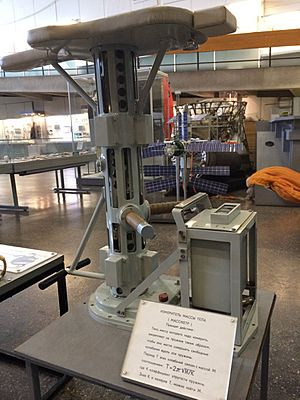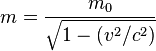Mass facts for kids

Kids Encyclopedia FactsMassmeter, a device for measuring the inertial mass of an astronaut in weightlessness. The mass is calculated via the oscillation period for a spring with the astronaut attached (Tsiolkovsky State Museum of the History of Cosmonautics)

The mass of an object is a measure of an object's resistance to acceleration, sometimes also called "inertia". A mountain has typically more mass than a rock, for instance. Mass should not be confused with the related but quite different concept of weight. A large mass like the Earth will attract a small mass like a human being with enough force to keep the human being from floating away. "Mass attraction" is another word for gravity, a force that exists between all matter.

Units of mass

The unit of mass in the International System of Units is the kilogram, which is represented by the symbol 'kg'. Fractions and multiples of this basic unit include the gram (one thousandth of a kg, symbol 'g') and the tonne (one thousand kg), amongst many others.

In some fields or applications, it is convenient to use different units to simplify the discussions or writings. For instance,

• Atomic physicists deal with the tiny masses of individual atoms and measure them in atomic mass units.
• Jewelers normally work with small gems and precious stones where masses are traditionally measured in carats, which correspond to 200 mg or 0.2 g.
• The masses of stars are very large and are sometimes expressed in units of Solar masses.

Traditional units are still in encountered in some countries: Imperial units such as the ounce or the pound were in widespread use within the British Empire. Some of them are still popular in the United States, which also uses units like the short ton ton (2,000 pounds) and the long ton (2,240 pounds), not to be confused with the metric tonne (1,000 kg).

Conservation of mass and relativity

Mass is an intrinsic property of the object: it does not depend on its volume, or position in space, for instance. For a long time (at least since the works of Antoine Lavoisier in the second half of the eighteen century), it has been known that the sum of the masses of objects that interact or of the chemicals that react remain conserved throughout these processes. This remains an excellent approximation for everyday life and even most laboratory work.

However, Einstein has shown through his special theory of relativity that the mass m of an object moving at speed v with respect to an observer must be higher than the mass of the same object observed at rest m0 with respect to the observer. The applicable formula is$m=\frac{m_0}{\sqrt{1 - (v^2/c^2)}}$

where c stands for the speed of light. This change in mass is only important when the speed of the object with respect to the observer becomes a large fraction of c.

Images for kidsMass Facts for Kids. Kiddle Encyclopedia.